# How to Add a User-Defined Table Function to Excel

Finaquant’s Excel add-in (Finaquant in Excel) comes with a list of table-valued functions like Table Arithmetics, Combine Tables, Filter Table, Aggregate Table, Distribute Table, and so on.

You can however extend this list with your own user-defined table functions. Following steps will show how you can do this by creating a simple and practical table function:
Get Price TableThis table function obtains a price table as output from the given input tables for product costs and margins. Note that with table function we mean a function whose input and output parameters are data tables.

When you add a table function to Excel as explained in the steps below, the new function will appear as a new item in the Command Menu named Table Functions: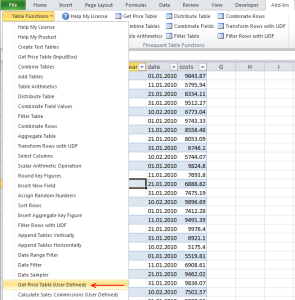And this is how the table calculation is performed in our example Get Price Table: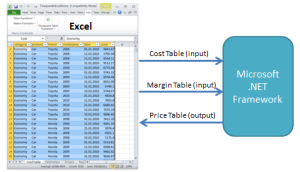In our example here (Get Price Table), all the input and output tables (ListObjects in Excel) are read from or written to Excel. The more general schema can be depicted as follows: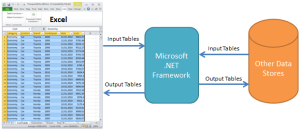As shown above, a user-defined table function could be written such that some data tables could be fetched from (or stored into) databases like MS SQL or MySQL.

For example, in a rule-based calculation, all the input tables that define the rules of the calculation (like contracts) can be kept in Excel sheets like a cockpit (as User Interface), and the bulk of the input/output data required for the calculation could be stored in an external database.

Let’s return back to our example. These are the steps required for adding the user-defined table function Get Price Table to Excel (for .NET developers):

 Download Description FinaquantInExcel_betaXXX.zip Zip package with Excel add-in (xll file) and Visual Studio project for developing the add-in (open source) IntroToFinaquantInExcel.pdf Introduction to Table-Valued Functions in Excel (add-in); a Visual Guide with table function examples and developer notes.

### 1) Download and open Visual Studio project named Finaquant in Excel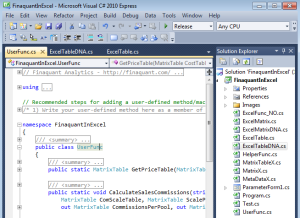Make sure that project build configuration is selected as Release (i.e. not Debug), and two files that you need for the Excel add-in are in the project folder bin/Release: `FinaquantInExcel_beta.dna` and `FinaquantInExcel_beta.xll`

You will realize that all the code is written in C# (c sharp) in this Visual Studio project. If you want to develop in another .NET language like VB.NET (Visual Basic for .NET) you can convert the C# code to the language you choose using tools like:UserFunc is the class where user-defined table functions are added as new methods.

UserFunc.GetPriceTable (C# code)
```/// <summary> /// Get price table with costs, margins and prices according to given cost and margin tables. /// </summary> /// <param name="CostTable">Cost table with key figure named "costs"</param> /// <param name="MarginTable">Margin table with key figure named "margin"</param> /// <returns>Price table</returns> public static MatrixTable GetPriceTable(MatrixTable CostTable, MatrixTable MarginTable) { // check inputs if (CostTable == null || CostTable.IsEmpty || CostTable.RowCount == 0) throw new Exception("GetPriceTable: Null or empty input table CostTable\n");   if (MarginTable == null || MarginTable.IsEmpty || MarginTable.RowCount == 0) throw new Exception("GetPriceTable: Null or empty input table MarginTable\n");   // check if CostTable has a key figure named "costs" if (! TextVector.IfValueFoundInSet("costs", CostTable.KeyFigureFields)) throw new Exception("GetPriceTable: CostTable must have a key figure named costs\n");   // check if MarginTable has a key figure named "margin" if (!TextVector.IfValueFoundInSet("margin", MarginTable.KeyFigureFields)) throw new Exception("GetPriceTable: MarginTable must have a key figure named margin\n");   // input checks OK, continue.. try { // CostTable: Exclude all key figures other than "costs" (only "costs" is required) MatrixTable CostTbl = MatrixTable.ExcludeColumns(CostTable, TextVector.SetDifference(CostTable.KeyFigureFields, new TextVector(new string[] { "costs" })));   // MarginTable: Exclude all key figures other than "margin" (only "margin" is required) MatrixTable MarginTbl = MatrixTable.ExcludeColumns(MarginTable, TextVector.SetDifference(MarginTable.KeyFigureFields, new TextVector(new string[] { "margin" })));   // Get Price Table MatrixTable PriceTbl = MatrixTable.MultiplySelectedKeyFigures( CostTbl, MarginTbl + 1, "costs", "margin", "price", JokerMatchesAllvalues: true);   // Combine MarginTbl to see margins in resultant price table PriceTbl = MatrixTable.CombineTables(PriceTbl, MarginTbl);   return PriceTbl; } catch (Exception ex) { throw new Exception("GetPriceTable: " + ex.Message + "\n"); } }```

### 3) Add two enveloper methods to class ExcelTable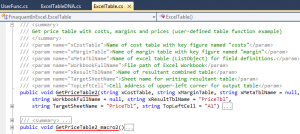First enveloper method `GetPriceTable2` defines all the input and output parameters and calls your user-defined function `UserFunc.GetPriceTable`. This method can directly be called from VBA Excel.

ExcelTable.GetPriceTable2 (C# code)
```/// <summary> /// Get price table with costs, margins and prices (user-defined table function example) /// </summary> /// <param name="xCostTable">Name of cost table with key figure named "costs"</param> /// <param name="xMarginTable">Name of margin table with key figure named "margin"</param> /// <param name="xMetaTblName">Name of excel table (ListObject) for field definitions.</param> /// <param name="WorkbookFullName">File path of Excel Workbook</param> /// <param name="xResultTblName">Name of resultant combined table</param> /// <param name="TargetSheetName">Sheet name for writing resultant table</param> /// <param name="TopLeftCell">Cell address of upper-left corner for output table</param> public void GetPriceTable2(string xCostTable, string xMarginTable, string xMetaTblName = null, string WorkbookFullName = null, string xResultTblName = "PriceTbl", string TargetSheetName = "PriceTbl", string TopLeftCell = "A1") { // Typical flow of calculation:   // Step 0: Get current application and active workbook Excel.Application xlApp = ExcelFunc_NO.GetExcelApplicationInstance(); Excel.Workbook wbook;   if (WorkbookFullName == null || WorkbookFullName == String.Empty) wbook = xlApp.ActiveWorkbook; else wbook = ExcelFunc_NO.GetWorkbookByFullName(xlApp, WorkbookFullName);   // Step 1: Get ListObjects XTable xTbl1 = ExcelFunc_NO.GetListObject(wbook, xCostTable); XTable xTbl2 = ExcelFunc_NO.GetListObject(wbook, xMarginTable);   XTable xMetaTbl = null; if (xMetaTblName != null && xMetaTblName != String.Empty) xMetaTbl = ExcelFunc_NO.GetListObject(wbook, xMetaTblName);   // Step 2: Get meta data with field definitions MetaDataX mdx = new MetaDataX(); if (xMetaTblName != null && xMetaTblName != String.Empty) mdx.ReadFieldsFromExcelTable(xMetaTbl); MetaData md = mdx.metaData;   // Step 3: Read excel tables (inputs) into MatrixTable objects MatrixTable Tbl1 = ExcelToMatrixTable(xTbl1, mdx).matrixTable; MatrixTable Tbl2 = ExcelToMatrixTable(xTbl2, mdx).matrixTable;   // Step 4: Generate resultant (output) tables with table functions MatrixTable PriceTbl = UserFunc.GetPriceTable(Tbl1, Tbl2);   // Step 5: Write output tables (in this case CombinedTbl) into excel tables Excel.Worksheet wsheet = ExcelFunc_NO.GetWorksheet(wbook, TargetSheetName, AddSheetIfNotFound: true);   MatrixTableToExcel(new MatrixTableX(PriceTbl), wsheet, xResultTblName, TopLeftCell); wsheet.Activate(); }```

Second enveloper method `GetPriceTable2 _macro2` reads all the input parameters from a windows form (see the related step below for adding the parameter form) and feeds them to the first method `GetPriceTable2` for execution.

ExcelTable.GetPriceTable2 _macro2 (C# code)
```/// <summary> /// Get price table with costs, margins and prices (user-defined table function example) /// </summary> public void GetPriceTable2_macro2() { try { // get current excel application Excel.Application xlapp = ExcelFunc_NO.GetExcelApplicationInstance();   // get active workbook Excel.Workbook wbook = xlapp.ActiveWorkbook;   // set global form parameters ParameterForm1.wbook_st = wbook; ParameterForm1.md_st = new MetaData();   string FormTitle = "Parameter Form for GetPriceTable2"; string FuncTitle = "Get Price Table"; string FuncDescr = "Get price table with costs, margins and prices. " + "Cost table must contain a key figure named costs, and margin table must contain a key figure named margin. " + "Resultant price table contains all three key figures: costs, margin and price";   using (ParameterForm1 myform = new ParameterForm1()) { myform.PrepForm_GetPriceTable2(FormTitle, FuncTitle, FuncDescr, "PriceTbl", "PriceTbl", "A1"); myform.ShowDialog(); }   // return if cancel button is pressed if (ParameterForm1.IfCancel) return;   // assign parameter values string xTb1Name = ParameterForm1.xTable1_st; string xTbl2Name = ParameterForm1.xTable2_st;   string xOutTable = ParameterForm1.xOutTable1_st; string SheetName = ParameterForm1.SheetName_st; string TopLeftCell = ParameterForm1.TopLeftCell_st;   // combine tables GetPriceTable2(xTb1Name, xTbl2Name, xResultTblName: xOutTable, TargetSheetName: SheetName, TopLeftCell: TopLeftCell); } catch (Exception ex) { MessageBox.Show("GetPriceTable2: " + ex.Message + "\n"); } }```

### 4) Add a Method to class ExcelTableDNA for Command Menu Item in Excel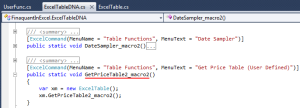This method (`GetPriceTable2_macro2`) in class ExcelTableDNA attributed with `ExcelCommand(...)` is necessary for inserting a new command item into the Command Menu in Excel (see below). The order of this method within the class ExcelTableDNA determines the order of the corresponding command item in Excel.

ExcelTableDNA.GetPriceTable2_macro2 (C# code)
```/// <summary> /// Get price table with costs, margins and prices (user-defined table function example) /// </summary> [ExcelCommand(MenuName = "Table Functions", MenuText = "Get Price Table (User Defined)")] public static void GetPriceTable2_macro2() { var xm = new ExcelTable(); xm.GetPriceTable2_macro2(); }```Add a new method to ParameterForm1 which prepares the parameter form for your table function. This parameter form will open to collect all the required input parameters when the user presses the corresponding command menu item (Get Price Table) in Excel.

ParameterForm1.PrepForm_GetPriceTable2 (C# code)
```// Prepare parameter form for get price table (example for user-defined table function) internal void PrepForm_GetPriceTable2(string FormTitle, string FuncTitle, string FuncDescr, string xOutTable, string SheetName, string TopLeftCell) { // init form this.Text = FormTitle + formbrand; this.FuncTitle.Text = FuncTitle; this.FuncDescription.Text = FuncDescr; int CTR = 0; // number of form elements like combo boxes, text boxes etc   // get list of all excel tables string[] TableList; ExcelFunc_NO.GetAllExcelTables(wbook_st, out TableList);   // get list of all worksheets string[] sheets = ExcelFunc_NO.GetAllSheetNames(wbook_st);   // initiate combo boxes if (TableList != null && TableList.Count() > 0) { // xTable1 AddComboBox(this.xTable1, Col2Start, ++CTR * LineWidth + ImageWidth, ComboBoxStyle.DropDownList, true, TableList); AddLabel(this.xTable1Lable, "Select Cost Table", Col1Start, CTR * LineWidth + ImageWidth, LableLen);   // xTable2 AddComboBox(this.xTable2, Col2Start, ++CTR * LineWidth + ImageWidth, ComboBoxStyle.DropDownList, true, TableList); AddLabel(this.xTable2Lable, "Select Margin Table", Col1Start, CTR * LineWidth + ImageWidth, LableLen); } else { this.RedWarning.Text = "No table was found in excel workbook!"; return; }   // xOutTable1 AddTextBox(this.xOutTable1, Col2Start, ++CTR * LineWidth + ImageWidth, 100, 20, xOutTable); AddLabel(this.xOutTable1Lable, "Enter name of output table", Col1Start, CTR * LineWidth + ImageWidth, LableLen);   // SheetName AddComboBox(this.SheetName, Col2Start, ++CTR * LineWidth + ImageWidth, ComboBoxStyle.DropDown, true, sheets, SheetName); AddLabel(this.SheetNameLable, "Select/Enter sheet name for output", Col1Start, CTR * LineWidth + ImageWidth, LableLen);   // text box (UpperLeftCell) AddTextBox(this.TopLeftCell, Col2Start, ++CTR * LineWidth + ImageWidth, 100, 20, TopLeftCell); AddLabel(this.TopLeftCellLable, "Address of upper-left cell for output", Col1Start, CTR * LineWidth + ImageWidth, LableLen);   // OK button AddButton(this.button_OK, Col2Start, ++CTR * LineWidth + ImageWidth, 75, 23, "OK", "OK"); this.button_OK.Click += new System.EventHandler(this.GetPriceTable2_OKbutton);   // CANCEL button AddButton(this.button_Cancel, Col2Start + 90, CTR * LineWidth + ImageWidth, 75, 23, "CANCEL", "CANCEL"); this.button_Cancel.Click += new System.EventHandler(this.button_Cancel_Click);   // form size this.Size = new System.Drawing.Size(FormWidth, ImageWidth + CTR * LineWidth + BottomMargin); }```

You may also need to add some event handlers that are specific to this windows form.

ParameterForm1.GetPriceTable2_OKbutton (C# code)
```private void GetPriceTable2_OKbutton(object sender, EventArgs e) { // validate input if (this.xTable1.SelectedItem == null) { this.RedWarning.Text = "A cost table must be selected!"; this.Refresh(); return; }   if (this.xTable2.SelectedItem == null) { this.RedWarning.Text = "A margin table must be selected!"; this.Refresh(); return; }   // check if cost table has a key figure named "costs" string InTbl1 = this.xTable1.SelectedItem.ToString(); TableFields tfields1 = ExcelFunc_NO.ReadTableFieldsFromExcel(InTbl1, md_st);   if (! TextVector.IfValueFoundInSet("costs", tfields1.KeyFigures)) { this.RedWarning.Text = "Selected cost table must contain a key figure named costs"; this.Refresh(); return; }   // check if margin table has a key figure named "margin" string InTbl2 = this.xTable2.SelectedItem.ToString(); TableFields tfields2 = ExcelFunc_NO.ReadTableFieldsFromExcel(InTbl2, md_st);   if (!TextVector.IfValueFoundInSet("margin", tfields2.KeyFigures)) { this.RedWarning.Text = "Selected margin table must contain a key figure named margin"; this.Refresh(); return; }   if (this.xOutTable1.Text == null || this.xOutTable1.Text.Trim() == "") { this.RedWarning.Text = "Name of output table must be entered!"; this.Refresh(); return; }   if (this.SheetName.SelectedItem == null && (this.SheetName.Text == null || this.SheetName.Text.Trim() == "")) { this.RedWarning.Text = "A worksheet for output table must be selected or entered!"; this.Refresh(); return; }   if (this.TopLeftCell.Text == null || this.TopLeftCell.Text.Trim() == "") { this.RedWarning.Text = "A upper-left cell address like A1 for output table must be entered!"; this.Refresh(); return; }   // checks OK, set static variables xTable1_st = this.xTable1.SelectedItem.ToString(); xTable2_st = this.xTable2.SelectedItem.ToString();   xOutTable1_st = this.xOutTable1.Text.Trim();   if (this.SheetName.SelectedItem == null) SheetName_st = this.SheetName.Text.Trim(); else SheetName_st = this.SheetName.SelectedItem.ToString();   TopLeftCell_st = this.TopLeftCell.Text.Trim(); IfCancel = false;   // close form this.Close(); }```

### 6) Rebuild (compile) the project and install add-in in Excel

Now that you have finished the code development you can add the updated add-in to Excel. Remember that your updated Excel add-in is in folder Release/bin: `FinaquantInExcel_beta.xll`

See add-in’s product page (Finaquant in Excel) for installation steps. Check if your table function appears in the Command Menu named Table Functions in Excel under the tab named Add-ins.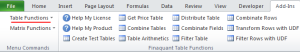You need to update the XML-formatted DNA file `FinaquantInExcel_beta.dna` in folder Release/bin if you want to see a command button (like Table Arithmetics above) in the ribbon named Finaquant Table Functions.

Following XML code in the group with id = Table Functions in `FinaquantInExcel_beta.dna` will add a command button for the table function Get Price Table:

``` <group id="TableFunctions" label="Finaquant Table Functions" insertAfterMso="GroupMenuCommands"> ... <button id="GetPriceTable2_macro2" tag="Get Price Table" onAction="RunTagMacro" label="&amp;Get Price Table" screentip="Obtain Price Table from Cost and Margin tables(Example UDF)" size="normal" imageMso="TableInsertDialog" /> ... </group>```

### 7) Pack your Excel Add-in into a single xll-file for distribution

You can easily produce a single excel add-in (xll) file for distribution using the utility application ExcelDNAPack.exe provided by ExcelDNA.

For this purpose, copy every dll file (.NET assemblies) required for the development of add-in into a folder, together with the DNA and xll files: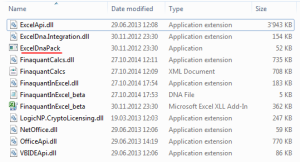Start Windows Command Prompt (START>cmd), change to current folder (cd ..) and enter following command to pack everything into a single xll file:
`ExcelDNAPack.exe FinaquantInExcel_beta.dna`

If everything goes well this command will produce an xll file named FinaquantInExcel_beta-packed.xll. You can rename this file as you like before distribution.

Note that your XML-formatted DNA file must contain all the required dll references for this packing process:

```<group id="TableFunctions" label="Finaquant Table Functions" insertAfterMso="GroupMenuCommands"> <DnaLibrary RuntimeVersion="v4.0" > <ExternalLibrary Path="FinaquantInExcel.dll" Pack="true" ComServer="true" /> <ExternalLibrary Path="FinaquantCalcs.dll" Pack="true" /> <ExternalLibrary Path="LogicNP.CryptoLicensing.dll" Pack="true" /> <ExternalLibrary Path="NetOffice.dll" Pack="true" /> <ExternalLibrary Path="ExcelApi.dll" Pack="true" /> <ExternalLibrary Path="OfficeApi.dll" Pack="true" /> <ExternalLibrary Path="VBIDEApi.dll" Pack="true" /> <CustomUI> ...```

## Final Notes

Although Finaquant’s excel add-in was developed specifically for exposing the table valued functions of the .NET library Finaquant Calcs to Excel, its open-source code provides .NET developers with a solid framework for integrating matrix or table valued functions of any .NET library with excel.

The development process for adding a new matrix function is very similar to the process explained here for table functions. The main difference is: Enveloper methods in the 3. and 4. steps above are added to the classes ExcelMatrix and ExcelMatrixDNA instead of ExcelTable and ExcelTableDNA.

Written By: Tunç Ali Kütükçüoglu

This entry was posted in Calculation engine and tagged , , . Bookmark the permalink.